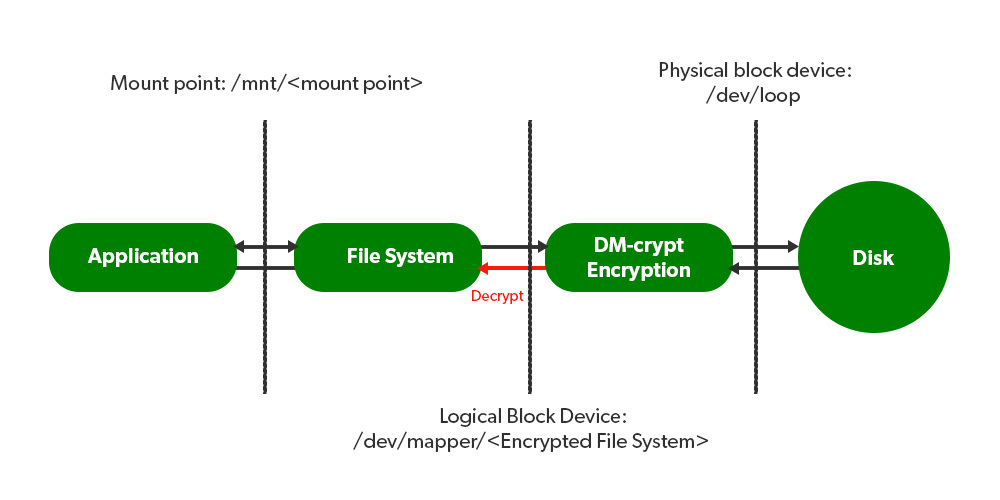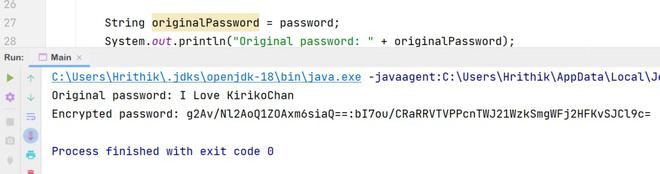Open in App
Not now

# Java Program to Encrypt Password in Configuration Files

• Last Updated : 09 May, 2022

Encryption is a system of mathematical algorithms that encodes user data so that only the intended recipient can read it. Encryption enhances the security of a message or file by scrambling the content. It is the most effective way to hide communication where the sender and the recipient hold the key to deciphering data. The scrambled and unreadable format is often known as the ciphertext format.

Illustration: Amazon EC2 configurationLet us first discuss the Need for Encryption. So, when a user sends a signup request with having username and password, then it gets transferred to the destination server across the internet or over wired or wireless connections where it gets stored in the database. Since there is no encryption a  hacker may intercept or steal the data in the middle when it is being transferred over the network. Also storing the plain text in the database is not secure at all hackers may break into the system and steal the passwords from the database.

## Encrypting Password in Configuration files

Password-Based Encryption in Java allows us to encrypt and decrypt a text by using a password. This basically means initializing a javax.crypto.Cipher with algorithm “AES/CBC/PKCS5Padding” and getting a key from javax.crypto.SecretKeyFactory with the “PBKDF2WithHmacSHA512” algorithm.

Let us first create a configuration file named config.properties file at the src/config.properties and put this inside the file:

`Password = I Love KirikoChan`
1. Now first instantiate the Properties class so that it can read the configuration file.
2.  Create an instance of the FileInputStream class by passing the path of the configuration file as an argument.
3.  Invoke the .load() method for loading the properties of the configuration file in the class, and this takes the FileInputStream instance as a parameter. It throws IllegalArgumentException if this input stream contains a malformed Unicode escape sequence and IOException if an error occurred when reading from the input stream.
4. Now use the .getProperty() method to search for the property with the specified key from the list of properties in the configuration file. It returns null if it is unable to find the properties.
5. Now create a Salt with any random String to add to the password string. A cryptographic salt is made up of random bits added to each password instance before its hashing. Salts create unique passwords even in the instance of two users choosing the same passwords. Salts help us mitigate hash table attacks by forcing attackers to re-compute them using the salts for each user.
6. Now call the .generateSecretKey() is a user-defined method that returns the SecretKeySpec key. This key is used to encrypt as well as decrypt the password. The .generateSecretKey() method is defined in the user-defined secretKey class.

Below is the implementation of the Encryption.java class file :

## Java

 `// Java Program to Implement Encryption Class  ` ` `  `package` `Exploit.org; ` ` `  `// Importing required classes  ` `import` `java.io.FileInputStream; ` `import` `java.io.IOException; ` `import` `java.security.GeneralSecurityException; ` `import` `java.util.Properties; ` `import` `javax.crypto.spec.SecretKeySpec; ` ` `  `// Class  ` `public` `class` `Encryption { ` ` `  `    ``// Main driver method  ` `    ``public` `static` `void` `main(String[] args) ` `        ``throws` `IOException, GeneralSecurityException ` `    ``{ ` `        ``Properties properties = ``new` `Properties(); ` `        ``FileInputStream fileInputStream ` `            ``= ``new` `FileInputStream(``"src/config.properties"``); ` `        ``properties.load(fileInputStream); ` `        ``String password ` `            ``= properties.getProperty(``"Password"``); ` ` `  `        ``if` `(password == ``null``) { ` `            ``throw` `new` `IllegalArgumentException( ` `                ``"Parameter is not present in configuration file"``); ` `        ``} ` ` `  `        ``byte``[] salt = ``new` `String(``"622836429"``).getBytes(); ` `        ``int` `iterationCount = ``10000``; ` `        ``int` `keyLength = ``128``; ` `       `  `        ``secretKey object = ``new` `secretKey(); ` `        ``SecretKeySpec key = object.generateSecretKey( ` `            ``password.toCharArray(), salt, iterationCount, ` `            ``keyLength); ` ` `  `        ``String originalPassword = password; ` `        ``System.out.println(``"Original password: "` `                           ``+ originalPassword); ` `        ``String encryptedPassword ` `            ``= object.encrypt(originalPassword, key); ` `       `  `        ``System.out.println(``"Encrypted password: "` `                           ``+ encryptedPassword); ` `    ``} ` `}`

Now let’s create the secretKey class that contains two method .generateSecretKey() and .encrypt().

1. The .generateSecretKey() is a function that takes parameters password, salt, iteration count, and key length.  We use the iteration count as the number of iterations that an Algorithm should take. The key length variable is the length of the key that we ultimately need to derive. This method throws NoSuchAlgorithmException and InvalidKeySpecException exceptions.
2. The .getInstance() method takes the standard name of the requested secret-key algorithm and returns the new SecretKeyFactory object.
3. Now we create an instance of PBEKeySpec. The constructor takes a password, salt, iteration count, and to-be-derived key length for generating PBEKey of variable-key-size PBE ciphers.
4. The encrypt method takes two parameters, the data to be encrypted and the key. This method throws two exceptions GeneralSecurityException and UnsupportedEncodingException.
5. The .init() method initializes the Cipher for one of the following four operations: encryption, decryption, key wrapping, or key unwrapping depending on the operation mode value.
6. We also need a  .base64Encoder(byte[] bytes) method. It is a private method that encodes the specified byte array into a string using the Base64 encoding scheme.

Example:

## Java

 `// Java Program to Illustrate Class that Contains ` ` `  `package` `Exploit.org; ` ` `  `// Importing required classes  ` `import` `java.io.UnsupportedEncodingException; ` `import` `java.security.AlgorithmParameters; ` `import` `java.security.GeneralSecurityException; ` `import` `java.security.NoSuchAlgorithmException; ` `import` `java.security.spec.InvalidKeySpecException; ` `import` `java.util.Base64; ` `import` `javax.crypto.Cipher; ` `import` `javax.crypto.SecretKey; ` `import` `javax.crypto.SecretKeyFactory; ` `import` `javax.crypto.spec.IvParameterSpec; ` `import` `javax.crypto.spec.PBEKeySpec; ` `import` `javax.crypto.spec.SecretKeySpec; ` ` `  `// Class  ` `public` `class` `secretKey { ` `   `  `    ``// Method   ` `    ``public` `SecretKeySpec ` `    ``generateSecretKey(``char``[] password, ``byte``[] salt, ` `                      ``int` `iterationCount, ``int` `keyLength) ` `        ``throws` `NoSuchAlgorithmException, ` `               ``InvalidKeySpecException ` `    ``{ ` `        ``SecretKeyFactory keyFactory ` `            ``= SecretKeyFactory.getInstance( ` `                ``"PBKDF2WithHmacSHA512"``); ` `        ``PBEKeySpec keySpec = ``new` `PBEKeySpec( ` `            ``password, salt, iterationCount, keyLength); ` `        ``SecretKey tempKey ` `            ``= keyFactory.generateSecret(keySpec); ` `        ``return` `new` `SecretKeySpec(tempKey.getEncoded(), ` `                                 ``"AES"``); ` `    ``} ` ` `  `    ``// Method  ` `    ``private` `String base64Encoder(``byte``[] bytes) ` `    ``{ ` `        ``return` `Base64.getEncoder().encodeToString(bytes); ` `    ``} ` ` `  `    ``// Method  ` `    ``public` `String encrypt(String dataToEncrypt, ` `                          ``SecretKeySpec key) ` `        ``throws` `GeneralSecurityException, ` `               ``UnsupportedEncodingException ` `    ``{ ` `        ``Cipher pbeCipher ` `            ``= Cipher.getInstance(``"AES/CBC/PKCS5Padding"``); ` `        ``pbeCipher.init(Cipher.ENCRYPT_MODE, key); ` `         `  `                 ``AlgorithmParameters parameters ` `            ``= pbeCipher.getParameters(); ` `         `  `                 ``IvParameterSpec ivParameterSpec ` `            ``= parameters.getParameterSpec( ` `                ``IvParameterSpec.``class``); ` `         `  `                 ``byte``[] cryptoText = pbeCipher.doFinal( ` `            ``dataToEncrypt.getBytes(``"UTF-8"``)); ` `         `  `                 ``byte``[] iv = ivParameterSpec.getIV(); ` `        ``return` `base64Encoder(iv) + ``":"` `            ``+ base64Encoder(cryptoText); ` `    ``} ` `}`

Output: Above code snippetsOutput

Let us finally discuss the constructors and methods used in the above two class files that are ciphers and as follows:

My Personal Notes arrow_drop_up
Related Articles